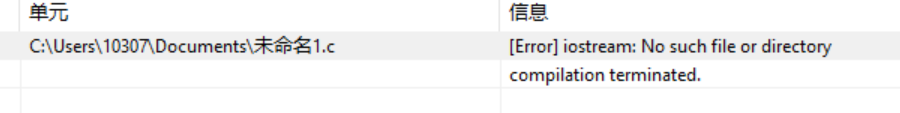#include
#include
#include
#include
using namespace std;
typedef struct Infor
{
char name; char infor;
}Infor;

typedef struct
{

int arcs;

int vexnum,arcnum;

}Mgraph;

typedef struct
{

int LocateVex(Mgraph &G,char a)
{
for(int i=0;i<G.vexnum;i++)
{ if(strcmp(G.vexs[i].name,a)==0)

{ return i; }
}
cout<<"输入有误!"<<endl; return -1;
}

{
ifstream inFile("graph.txt");
char v1,v2; int i,j,k,w; inFile>>G.vexnum>>G.arcnum;
for(i=0;i {
inFile>>G.vexs[i].name;

inFile>>G.vexs[i].infor;
}
for(i=0;i {
for(int j=0;j {
G.arcs[i][j]=10000;
}
}
for(k=0;k {
inFile>>v1>>v2>>w;

i=LocateVex(G,v1);

j=LocateVex(G,v2); if(i==j)

{

G.arcs[i][j]=0;

}

else

{

G.arcs[i][j]=w;

G.arcs[j][i]=G.arcs[i][j];

}
}
for(int m=0;m {
}
}

void infor(Mgraph &G)
{
char a;
int b=1;
while(b)
{
for(int i=0;i {
cout }
cout cin>>a;
for(i=0;i<G.vexnum;i++)
{
if(strcmp(G.vexs[i].name,a)==0)
{
cout<<G.vexs[i].infor<<endl;
b=0;
}

}
if(b!=0)
{
cout<<"输入错误请重新输入！！"< }
cout cin>>b;
}
}

void ShortestPath (Mgraph G)//最短路径
{
char a,d;
int b=1,i,j,v,v0,w;
int Dist,S,Path;
int n=G.vexnum;
while(b)
{
for(i=0;i {
cout }
for(i=0;i {
Dist[i]=9999;
S[i]=0;
Path[i]=-1;
}
cout cin>>a;
cin>>d;
v0=LocateVex(G,a);
j=LocateVex(G,d);
for(v=0;v {
S[v]=0;
Dist[v]=G.arcs[v0][v];
if(Dist[v] Path[v]=v0;
else
Path[v]=-1;//v无前趋
}
Dist[v0]=0;
S[v0]=1;
for(i=1;i {
int min=9999;
for(w=0;w if(!S[w]&&Dist[w] {
v=w;
min=Dist[w];
}//w顶点离v1顶点更近
S[v]=1;
for(w=0;w if(!S[w]&&(Dist[v]+G.arcs[v][w] {
Dist[w]=Dist[v]+G.arcs[v][w];
Path[w]=v;
}//end if
}//end for
cout cout cout int f=Path[j],e;
i=0;
while(f!=-1)
{
e[i]=f;
f=Path[f];
i++;
}
for(v=i-1;v>=0;v--)
{
cout<";
}
cout< cout cin>>b;
}
}

void reception(Mgraph &G)//前台
{

int n;
while(1)
{
system("cls");//清屏
cout<<"*********************欢迎使用前台系统************************"< cout cout cout cin>>n;
switch(n)
{
case 1:
infor(G);
break;
case 2:
ShortestPath (G);
break;
case 0:
return;
break;
default:
cout<<"您的输入有误,任意键继续..."<<endl;
getch();

}
}
}
{
int i,j;
ofstream outFile("graph.txt");
if(!outFile)
{
cerr<<"cannot open my.txt"<<endl;
exit(1);
}
outFile<<G.vexnum<<" "<<G.arcnum<<endl;
for(i=0;i<G.vexnum;i++)
{
outFile<<G.vexs[i].name<<" "<<G.vexs[i].infor<<endl;
}

for(i=0;i {
for(j=0;j {
if(G.arcs[i][j]!=10000)
{
outFile G.arcs[j][i]=10000;
}
}
}
Creategraph(G,pw);
}
{
char a;
int b=1,i;
while(b)
{
for(i=0;i {
cout }
cout cin>>a;
for(i=0;i {
if(strcmp(a,G.vexs[i].name)==0)
{
cout cout cin>>G.vexs[i].infor;
cout<<"修改成功!!!!"< b=0;
}
}
if(b!=0)
{
cout }
cout int c;
cin>>c;
if(c==1)
{
WriteTXT(G,pw);
}
cout<<"返回后台系统按0,继续修改按1"< cin>>b;
}
}
{
char a;
int b=1,i,j,k;
while(b)
{
for(i=0;i {
cout }
cout cin>>a;
for(i=0;i {
if(strcmp(a,G.vexs[i].name)==0)
{
for(j=i;j {
G.vexs[j]=G.vexs[j+1];
for(k=0;k G.arcs[k][j]=G.arcs[k][j+1];
}
for(j=i;j {
for(k=0;k G.arcs[j][k]=G.arcs[j+1][k];
}
G.vexnum--;
G.arcnum=0;
for(i=0;i {
for(j=0;j {
if(G.arcs[i][j]!=10000)
G.arcnum++;
}
}
G.arcnum=G.arcnum/2;
b=0;
cout }
}
if(b!=0)
{
cout }
cout int c;
cin>>c;
if(c==1)
{
WriteTXT(G,pw);
}
cout<<"返回后台系统按0,继续删除按1"< cin>>b;
}
}

{
char a,d;
int b=1,i,j;
while(b)
{
for(i=0;i {
for(j=0;j {
if(G.arcs[i][j]!=10000)
{
cout }
}
}
cout cin>>a;
cin>>d;
i=LocateVex(G,a);
j=LocateVex(G,d);
if(G.arcs[i][j]!=10000)
{
G.arcs[i][j]=10000;
G.arcs[j][i]=10000;
b=0;
cout<<"删除成功！！"< G.arcnum--;
}
if(b!=0)
{
cout }
cout int c;
cin>>c;
if(c==1)
{
WriteTXT(G,pw);
}
cout<<"返回后台系统按0,继续删除按1"< cin>>b;
}
}
///////////////////////////////////选作//////////////////////////

{

cout<<"请输入景点名称："< cin>>G.vexs[G.vexnum].name;
cout<<"请输入景点信息："< cin>>G.vexs[G.vexnum].infor;

for(int i=0;i<G.vexnum;i++)
G.arcs[G.vexnum][i]=10000;
for(i=0;i<G.vexnum;i++)
G.arcs[i][G.vexnum]=10000;

G.arcs[G.vexnum][G.vexnum]=0;

G.vexnum++;

cout<<"增加成功!"<<endl;
cout<<endl;
WriteTXT(G,pw);
system("pause");system("cls");
}

////////////////////////////////////////////////
{
char p;
cout<<"请输入6位密码："<<endl;
for(int e=0;e<6;e++)
{
p[e]=getch();
cout<<"*";
cout.flush();
}
cout<<endl;
for(e=0;e<6;e++)
{

}
cout<<endl;
return true;
}

{
int n;
while(1)
{
system("cls");
cout<<"*********************欢迎使用后台系统************************"< cout cout cout cout cout cin>>n;
switch(n)
{
case 1:
ChangeP(G,pw);
break;
case 2:
deleteP(G,pw);
break;
case 3:
deleteL(G,pw);
break;
case 4:
break;
case 0:
return;
break;
default:
cout<<"您的输入有误,任意键继续..."<<endl;
getch();

}
}
}

////////////////////////////////////////////////////////////////////////////////////////////////

void main()//主函数
{
Mgraph G;
Creategraph(G,pw);
int n,m=1;
while(m)
{
system("cls");
cout<<"*********************欢迎使用北林游览系统************************"< cout cout cout cin>>n;
switch(n)
{
case 1:
reception(G);
break;
case 2:
{
backstage(G,pw);//后台函数，并调用
}
else
cout<<"密码输入错误！！";
break;
case 0:
m=0;
break;
default:
cout<<"您的输入有误,任意键继续..."<<endl;
getch();
}
}
}1个回答

C语言不支持iostream，如果是C++，你应该用cpp作为后缀名，而不是c